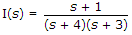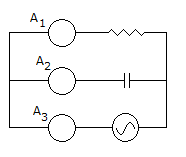# Electronics and Communication Engineering - Networks Analysis and Synthesis

21.

A cable has an insulation resistance of 1010 ohms. If the length is doubled, the insulation resistance will be

 A. 2 x 1010 Ω B. 0.5 x 1010 Ω C. 1010 Ω D. 4 x 1010 Ω

Explanation:

Insulation resistance of cable is inversely proportional to length.

22., i(t) =

 A. 3e-4t - e-5t B. 3e-4t - 2e-3t C. 2e-4t - 5e-2t D. 3e-4t - e-t

Explanation:i(t) = 3e-4t - 2e-3t

23.

In the figure shown, A1, A2, A3 are identical Ammeters. If A1 and A3 read 5 and 13 A respectively, reading of A2 will beA. 8 B. 13 A C. 18 D. 12 A

Explanation:

A2 = 132 - 2512 A.

24.

Which of the following pairs are correctly matched?

1. Brune's realisation : realisation with ideal transformer.
2. Cauer realisation : ladder realisation.
3. Bott Duffin realisation : with non ideal transformer.
Select the answer using the following codes:

 A. 1, 2 and 3 B. 2 and 3 C. 1 and 3 D. 1 and 2

Explanation:

Bott Duffins realisation does not use transformer.

25.

The synthesis of minimum function was suggested by

 A. O'Brune B. R. Richards C. Bott and Duffin D. none of the above